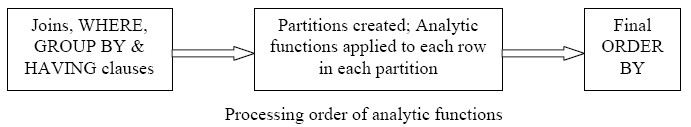# Function - Cumulative Function

## Example

### Oracle Sql

``````SELECT
SUM(SAL) OVER (ORDER BY DATE) CUMSUM,
SUM(SAL) OVER (PARTITION BY ACCOUNT ORDER BY DATE) CUMSUM_OVER_ACCOUNT,
FROM
...```
```

### R

With a R - Data Table

``````ledgerExpenseCumulative <- ledgerExpense[,.(amount=sum(amount)),keyby=.(account,date)
][,.(date=.SD\$date, amount=.SD\$amount,cumAmount=cumsum(.SD\$amount)),by=.(account)]```
```

where:

• the first pass calculate the sum of an account for a date and order the set
• the second pass calculate the cumulatif sum on the partition (.SD) by account

Recommended PagesSQL Function - Window Aggregate (Analytics function)

Windowing functions (known also as analytics) allow to compute: cumulative, moving, and aggregates. They are distinguished from ordinary SQL functions by the presence of an OVER clause. With...Time Serie - Moving Average (MA) - (Rolling|running)

(moving|rolling|running) average A moving average is commonly used with time series data to smooth out short-term fluctuations and highlight longer-term trends or cycles (Seasonality). Variations include:...